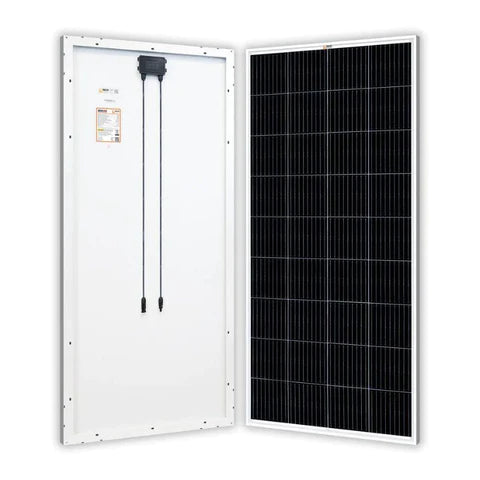Amp Draw Calculator - ShopSolar.com

# Amp Draw Calculator

Solar power doesn’t have to be complicated when you know the basic terminology and calculations. Do you want to calculate the amperage draw of your solar system? It’s really easy to do when you use our amp draw calculator.

If you’re new to solar energy, it may seem a bit daunting. But as the nation's number one source of solar power, we have the expertise to simplify your solar experience. With our simple explanations and easy-to-follow example, you’ll understand how to calculate amp draw in no time.

```// <![CDATA[
form_structure_1 = [[{
"dependencies": [{
"rule": "",
"complex": false,
"fields": [""]
}],
"form_identifier": "",
"name": "fieldname6",
"shortlabel": "",
"index": 0,
"ftype": "fCalculated",
"userhelp": "",
"audiotutorial": "",
"userhelpTooltip": false,
"tooltipIcon": false,
"csslayout": "",
"title": "Power Rating (watts)",
"predefined": "",
"required": false,
"exclude": false,
"size": "large",
"eq": "fieldname1*fieldname7",
"min": "",
"max": "",
"suffix": "",
"prefix": "",
"decimalsymbol": ".",
"groupingsymbol": "",
"currency": false,
"noEvalIfManual": true,
"formatDynamically": false,
"hidefield": false,
"fBuild": {},
"parent": ""
}, {
"dependencies": [{
"rule": "",
"complex": false,
"fields": [""]
}],
"form_identifier": "",
"name": "fieldname7",
"shortlabel": "",
"index": 1,
"ftype": "fCalculated",
"userhelp": "",
"audiotutorial": "",
"userhelpTooltip": false,
"tooltipIcon": false,
"csslayout": "",
"title": "Source Voltage (volts)",
"predefined": "",
"required": false,
"exclude": false,
"size": "large",
"eq": "fieldname6\/fieldname1",
"min": "",
"max": "",
"suffix": "",
"prefix": "",
"decimalsymbol": ".",
"groupingsymbol": "",
"currency": false,
"noEvalIfManual": true,
"formatDynamically": false,
"hidefield": false,
"fBuild": {},
"parent": ""
}, {
"dependencies": [{
"rule": "",
"complex": false,
"fields": [""]
}],
"form_identifier": "",
"name": "fieldname1",
"shortlabel": "",
"index": 2,
"ftype": "fCalculated",
"userhelp": "",
"audiotutorial": "",
"userhelpTooltip": false,
"tooltipIcon": false,
"csslayout": "",
"title": "Amps Draw",
"predefined": "",
"required": false,
"exclude": false,
"size": "large",
"eq": "fieldname6\/fieldname7",
"min": "",
"max": "",
"suffix": "",
"prefix": "",
"decimalsymbol": ".",
"groupingsymbol": "",
"currency": false,
"noEvalIfManual": true,
"formatDynamically": false,
"hidefield": false,
"fBuild": {},
"parent": ""
}, {
"form_identifier": "",
"name": "fieldname4",
"shortlabel": "",
"index": 3,
"ftype": "fButton",
"userhelp": "",
"audiotutorial": "",
"userhelpTooltip": false,
"tooltipIcon": false,
"csslayout": "col-md-6",
"sType": "calculate",
"sValue": "Calculate",
"sOnclick": "",
"sOnmousedown": "",
"sMultipage": false,
"fBuild": {},
"parent": ""
}, {
"form_identifier": "",
"name": "fieldname5",
"shortlabel": "",
"index": 4,
"ftype": "fButton",
"userhelp": "",
"audiotutorial": "",
"userhelpTooltip": false,
"tooltipIcon": false,
"csslayout": "col-md-6",
"sType": "reset",
"sValue": "Reset",
"sOnclick": "",
"sOnmousedown": "",
"sMultipage": false,
"fBuild": {},
"parent": ""
}], {
"0": {
"title": "Amps Draw Calculator",
"description": "",
"formlayout": "top_aligned",
"formtemplate": "cp_cff_clean",
"evalequations": 0,
"evalequationsevent": 2,
"autocomplete": 1,
"persistence": 0,
"customstyles": "",
"request_cost": "fieldname6",
"animate_form": 0
},
"formid": "cp_calculatedfieldsf_pform_1",
"setCache": true,
"cache": true
}];
// ]]>
```Before we get to what amperage draw is, and how to calculate it, let’s go over all the terminology used.

When calculating electricity usage, you need to factor in three things: volts, watts, and amps. These three measurements of electricity are useful for doing all sorts of calculations.

• The voltage/volts is the available electricity at the power sources. Knowing the volts reading is helpful. When you know the volts, you can convert voltage to amps.
• The amperes/amps are the amount of current flowing through the wires. When you know the amps you can convert amps to VA with a simple calculation.
• And the wattage/watts is the power produced by the electricity. Don’t know the watts? An ampere-to-watt converter calculator will give you the answer.

Amps are always useful to know. Once you’ve calculated the amps of a current, you can determine the solar cable size needed with an amp wire gauge calculator.

So, what is an amperage draw? It is the term given to describe the total current in amps delivered to a device. The amp draw is dependent on the source voltage and wattage rating of that device.

Amps are always said to be drawn, never pushed. Hence the term, amperage draw. Amps can also be expressed as milliamps. To convert amps to milliamps simply multiply the amps by 1,000. Some smaller appliances use milliamps, so it’s useful to know both units of measurement.

## Amp Usage CalculatorAn amp usage calculator or amp draw calculator is the calculation you can do to determine the amps used by your solar panel.

You need to divide the watts by the total number of volts available.

## Wattage rating/Source voltage

The result will be the maximum power current or amperage draw. And whether for solar systems or any other electrical setup, amp draw is calculated in the same way.

But to better explain how this works with solar panels, we’ll give you a real-world example using one of our products.

## Example of Amp Draw Calculation for Solar Panels

You’ve bought your solar panels and want to calculate the amp draw. It doesn’t matter whether they are traditional rigid residential solar panels or flexible marine solar panels. The next steps are the same.

• Look at the back of your solar panel or consult the manual for your panel’s specs.

Find the maximum rated power in watts, and the maximum power voltage, or Vmp. Every solar panel has these specs listed.The maximum power (Pmax) of this particular solar panel is 200 W and the maximum power voltage (Vmp) is 20.4 V

• We then divide the 200 watts by the 20.4 volts. You can use any calculator to do this calculation, even the one on your mobile phone.

200 / 20.4

The answer we get is 9.80

• The amp draw of this solar panel is 9.80 amps. That is the maximum power current of your solar panel.

## Conclusion

As you can see, calculating the maximum power current of a solar panel is easy once you know the formula. With our simple amp draw calculator, you can calculate the amp usage of any device.

You can also make use of our solar load calculator to determine your needs.

You can find all of the solar & electrical calculators we have created in order to help you plan, size and install your own solar power system here.

## Join the Solar Lab!